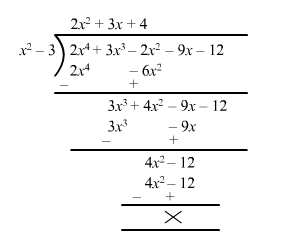# By actual division, show thatQuestion:

By actual division, show that $x^{2}-3$ is a factor of $2 x^{4}+3 x^{3}-2 x^{2}-9 x-12$

Solution:

Let $f(x)=2 x^{4}+3 x^{3}-2 x^{2}-9 x-12$ and $g(x)=x^{2}-3$Quotient $q(x)=2 x^{2}+3 x+4$

Remainder $r(x)=0$

Since, the remainder is 0 .

Hence, $x^{2}-3$ is a factor of $2 x^{4}+3 x^{3}-2 x^{2}-9 x-12$